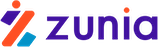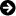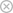Tuyển sinh 2023 dành cho Gen-Z# Specification Error

Chia sẻ: Hgiang Hgiang | Ngày: | Loại File: DOC | Số trang:1390
lượt xem
4

When constructing any regression model, we are always most interested in explaining what variables cause the dependent variable to change and by how much. This will always depend on a combination of economic theory; basic human behavior; and past experience.

Chủ đề:

## Nội dung Text: Specification Error

1. Nguyeãn Troïng Hoaøi Analytical Methods 9 1 Specification Error When constructing any regression model, we are always most interested in explaining what variables cause the dependent variable to change and by how much. This will always depend on a combination of economic theory; basic human behavior; and past experience. One of the assumptions of OLS is that the model is correctly specified. The specification error can be explained by these two aspects : - a) Missing / omitting relevant information / explanatory variables or from including irrelevant variables. b) Incorrect functional form. This lecture will discuss the following issues : which regressors should be included and / or excluded from a particular model. In other words, we will consider the following cases : - a) A regression model that excludes some important explanatory variables. b) A regression model that includes some irrelevant regressors. 1) Exclusion of relevant variables Suppose that we are interested in the following model : - Yi = β1 + β 2 X 2i + L + β K X Ki + β ( K + 1 ) X ( K +1) i + L + β( K + L ) X ( K + L ) i + εi The question is whether the set of L regressors - X( K + 1 ) + L + X( K + L ) - are important variables that should be included in the model. But because of a certain reason, we have to use the following model : - Yi = β1 + β 2 X 2i + L + β K X Ki + ε i For illustration, we can use a model with only two explanatory variables. The model with two explanatory variables is specified as follows : - True model Yi = β1 + β 2 X 2i + β 3 X 3i + ε i 9.1 Note: we assumed that X2 and X3 are the two important regressors that explain the dependent variable Y, that is, we expect that β 3 # 0. The model we use to estimate is as follows : - Estimation model Yi = β1 + β 2 X 2i + ε i 9.2 This means we have excluded an important regressor X3i. 1
2. Nguyeãn Troïng Hoaøi Analytical Methods 9 2 ˆ The LS estimator of β 2 is. ˆ β2 = ∑x Y 2i i 9.3 ∑x 2 2i Recall the lecture of Prof. Motahar in calculating the coefficient for regressor X2. Important consequences of excluding important explanatory variables ˆ a) E  β 2  ≠ ˆ β 2 and E  β 2  = β 2 if only if COV(X2,X3) = 0     To calculate the mathematical expectation of this estimate, we must substitute Yi with the formula for the true model, since our true model is 9.1 : - ( Yi = β 1 + β 2 X 2i + β 3 X 3i + ε i )  ˆ[ ] = E ∑ x E β2 2i  9.4  x 2i 2 ∑   ˆ[ ]= E β2 β2 + β3 ∑x X 2i 3i 9.5 ∑x 2 2i ∑x X 2i 3i = ∑x x 2i 3i 9.6 ∑x 2 2i ∑x 2 2i We can easily prove 9.5 and its numerator COV(X2,X3) ˆ b) β 2 is no longer explained as the direct effect (net) on the dependent variable Y. Notice that when omitting relevant variables, the estimated coefficient of the explanatory variable is insignificant in explaining the direct effect (net) on the dependent variable. We prove this as follows : - Recall the simple regression of Prof Motahar in defining the slope of Yi = β1 + β 2 X 2i + ε i β2 = ∑ ∧ x 2iYi 9.7 ∑x 2 2i So, if the simple regression is X 3i = β1 + β 22 X 2i + ε i the coefficient of X2 can also be defined by the expression, in which,the estimator is : - β 22 = ∑ ∧ x 2i X 3i 9.8 ∑x 2 2i 2
3. Nguyeãn Troïng Hoaøi Analytical Methods 9 3 This coefficient is the direct effect of X2 on X3 ∧  β2 =  ∑x ( 2i Yi = β1 + β 2 X 2i + β 3 X 3i + ε i )     ∑ x22i   n n n n n ∑1 xi  β1 + β 2 X i + β3 + ε i  i∑1 x2i   ∑x 2i X 2i ∑ x2i X 3i i∑1 x2iε i ˆ β2 = i=  n =β = 1 n + β2 i=1 n + β 3 i = 1n + =n ∑ xi 2 i =1 ∑ x2i 2 i =1 i =1 ∑x 2 2i ∑ x22i ∑ x22i i =1 i =1 n n n n Now notice that ∑ x i = ∑ ( Xi − X) = 0 vaø ∑ x i X i = ∑ x i2 as compared with : - i =1 i =1 i =1 i=1 n ∑x X i =1 i i n =1 ∑x i =1 2 i Thus, n n n ∑ x2i X 2i ∑ x2i X 3i ∑x 2i iε ˆ β2 = β2 i=1 +β i=1 + i=1 9.9 n 3 n n i =1 ∑x 2 2i ∑x i =1 2 2i ∑x i =1 2 2i And we also have : - n ∑x i=1 2i i ε / n = COV ( X 2 , ε ) = 0 According to infinite samples and OLS assumptions we ∧ ˆ have : - β 2 = β 2 + β 3 . β 22 9.10 Important meanings : ˆ Gross effect of X2 on Y in the model, β 2 equals the direct effect of X2 on Y (that ∧ ∧ is, β 2 in the true model) plus the indirect effect of X2 on Y (that is, β 3 . β 22 ). Thus, the estimated coefficient in the regression without X3 (and assuming that this ˆ variable is relevant), so then β 2 is insignificant in explaining a direct effect (net) on Y. We can graphically illustrate this and address some examples. 3
4. Nguyeãn Troïng Hoaøi Analytical Methods 9 4 This regression shows that HOUSING is explained quite well through GNP and INT.RATE. If we temporarily assume that this is the true model, we then regress HOUSING against GNP. 4
5. Nguyeãn Troïng Hoaøi Analytical Methods 9 5 We can conclude that this model excluded an important explanatory variable - INT.RATE (Observe how the coefficient of determination; the coefficient of GNP; and the standard error of the estimator of GNP change). Conduct another regression : INT.RATE on GNP 5
6. Nguyeãn Troïng Hoaøi Analytical Methods 9 6 Based on these three regression results, let us now consider what we have studied in 9.10. c) Variance of the estimate of the coefficient in the model is biased and thus tests on this hypothesis are invalid. ˆ 1 VAR  β 2  = σ 2 in the estimated model   ( ∑ x2i ) 2 9.11 but because β 3 # 0 and since we have assumed that X3 is an important and relevant factor in explaining Y, then : - ˆ[ ] = ( x )1(1 - r ) σ VAR β 2 2 9.12 ∑ 2 2i 2 23 9.11 is the variance in the estimated model and 9.12 is the variance when we assume β 3 # 0. It is obvious that : - ˆ VAR  β 2  = 1 [ ] = ( x )1(1 - r ) σ ˆ σ 2 < VAR β 2 2   (∑ x2i ) 2 ∑ 2 2i 2 23 9.14 6
7. Nguyeãn Troïng Hoaøi Analytical Methods 9 7 ∧ Therefore, the standard error of the estimator β 2 will be inaccurate (unstable, or biased), and thus the use of its standard error is inaccurate, too. As a result, any hypotheses testing will be invalid. From looking at the regression results, we will easily see that. For caution, we use the Wald test for a restricted model (an estimated model) and for an unrestricted model (a true model), based on the hypothesis that β 3 = 0. 2. Including irrelevant variables To analyze this case, we return again to the two-regressor model, only this time we assume that X3 does not relate to Y (that is β 3 = 0 ). In other words, X3 is irrelevant. True model Yi = β1 + β 2 X 2i + ε i Estimated model Yi = β1 + β 2 X 2i + β 3 X 3i + ε i The estimated model has the following criteria : - a) Estimators of other coefficients (except X3) are unbiased and consistent. Again, if we take the estimated coefficients and calculate their expectations : - 7
8. Nguyeãn Troïng Hoaøi Analytical Methods 9 8 ˆ (∑ Y x ) (∑ x ) - (∑ Y x ) (∑ x i 2i 2 3i i 3i 2i x 3i ) β2 = 9.15 (∑ x ) (∑ x ) - (∑ x x ) 2 2i 2 3i 2i 3i 2 Then substitute the true model for Yi and do some manipulation : - ˆ β2 = β2 (∑ x ) (∑ x ) - β ( ∑ x x ) 2 2i 2 3i 2 2i 3i 2 + (∑ ε x ) (∑ x ) - ( ∑ ε x )(∑ x i 2i 2 3i i 3i 2i x 3i ) 9.16 (∑ x ) (∑ x ) - (∑ x x ) 2 2i 2 3i 2i 3i 2 (∑ x ) (∑ x ) - ( ∑ x x ) 2 2i 2 3i 2i 3i 2 Clearly, the first term is β 2 and the second term zero expectation, so the estimator is unbiased. From looking at the second term of expression 9.16 we can find that : - ( Sε x2 / n)( S33 / n) - ( Sε x3 / n)( S 23 / n) ( S 22 / n)( S33 / n) - ( S23 / n ) 2 Since, as n is larger, then ( Sε x2 / n) and ( Sε x3 / n) converge to COV (ε , X) = 0. Hence, we find that this estimator has consistency. Now consider the coefficient of estimator for the variable that has been inappropriately included : - ˆ (∑ Y x ) (∑ x ) - (∑ Y x ) (∑ x i 3i 2 2i i 2i 2i x 3i ) β3 = (∑ x ) (∑ x ) - (∑ x x ) 2 2i 2 3i 2i 3i 2 Again, substitute the true model for Yi and do some manipulation : - ˆ (∑ ε x ) (∑ x ) - (∑ ε x ) (∑ x i 3i 2 2i i 2i 2i x 3i ) β3 = 0 + (∑ x ) (∑ x ) - (∑ x x ) 2 2i 2 3i 2i 3i 2 The expectation for this estimator is zero. b) Variances for the estimators are higher than for those excluding irrelevant variables, so those estimators are inefficient because the variance is not minimal. See expression 9.14. c) Variances of the estimators are unbiased so hypothesis testing is still valid. In conclusion : We find that when we include irrelevant variables, we get unbiased estimators for all of the coefficients, but the cost is that the minimum variances are larger than they would otherwise be. 8
9. Nguyeãn Troïng Hoaøi Analytical Methods 9 9 For example, for including irrelevant variables in the equation, we can add two more, such as population - POP - and unemployment - UNEMP - into the model : - Now examine the regression results, especially for the two new variables. Since we assume that the two new variables are irrelevant, we are going to do the Wald test on these. 9
10. Nguyeãn Troïng Hoaøi Analytical Methods 9 10 3) General – to – Simple Modeling Strategy The results that we have just established suggest that the general-to-simple modeling strategy is superior to the simple-to-general strategy. The steps are as follows : - [ Use economic theory, previous research, and experience to specify a general model (in this case “general” means a model that includes all possible relevant regressors). [ Estimate the model [ If any of the coefficients are statistically insignificant, omit the least significant one and re-estimate. Variables are eliminated one-by-one because of the effect of the elimination on the remaining variables. If the first regression shows two insignificant variables, and the least significant one is then omitted, this may increase the significance of the remaining one. [ From using the Wald Tests to test the final model (the restricted model), compare against the initial general model (the unrestricted model). 4) An application of modelling Strategy 10
11. Nguyeãn Troïng Hoaøi Analytical Methods 9 11 11
12. Nguyeãn Troïng Hoaøi Analytical Methods 9 12 12
13. Nguyeãn Troïng Hoaøi Analytical Methods 9 13 13